# Statements and open problems on decidable sets X \subseteq N that contain informal notions and refer to the current knowledge on X

### Full PDFcreative_2023_32_2_247_253

For a set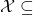whose infiniteness is false or unproven, we define which elements of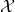are classified as known. No known setsatisfies Conditions~{\tt (1)-(4)} and is widely known in number theory or naturally defined, where this term has only informal meaning.
{\tt (1)}~A~known algorithm with no input returns an integer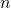satisfying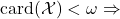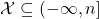.
{\tt (2)}~A~known algorithm for every \mbox{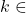} decides whether or not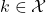.
{\tt (3)}~No known algorithm with no input returns the logical value of the statement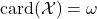.
{\tt (4)}~There are many elements of~and it is conjectured, though so far unproven, thatis infinite.
{\tt (5)}is naturally defined. The infiniteness ofis false or unproven.has the simplest definition among known sets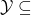with the same set of known elements.
The set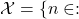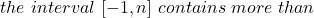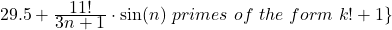satisfies Conditions~{\tt (1)-(5)} except the requirement thatis naturally defined.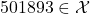. Condition~{\tt (1)} holds with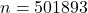.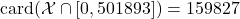.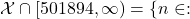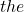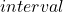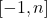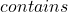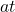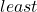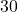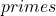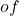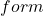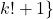. We present a table that shows satisfiable conjunctions of the form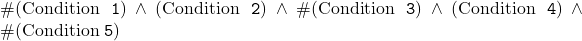, where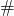denotes the negationor the absence of any symbol. No setwill satisfy Conditions~{\tt (1)-(4)} forever, if for every algorithm with no input,at some future day, a computer will be able to execute this algorithm in~second or less.